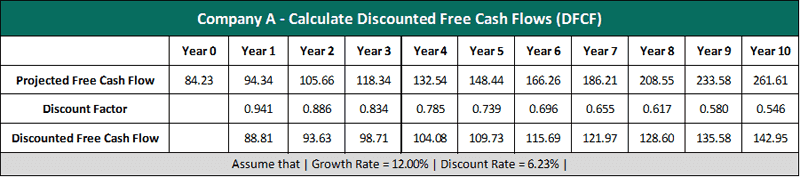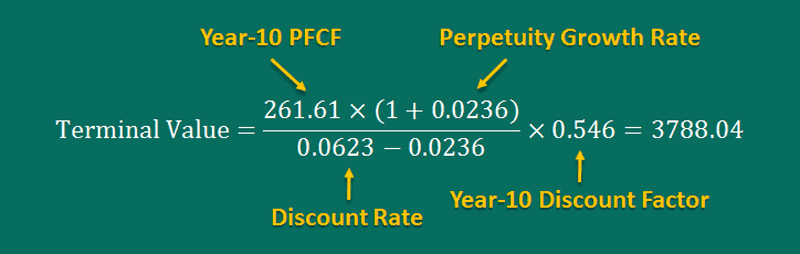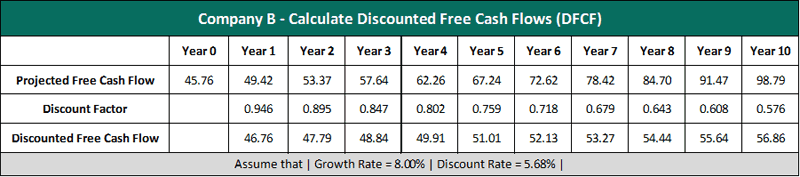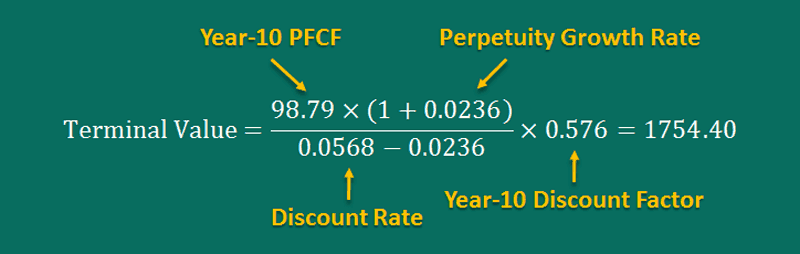3

# How to Calculate Terminal Value: The Most Comprehensive Guide! (Updated 2018)

This is an advanced﻿﻿ guide on how to calculate Terminal Value of a company with in-depth interpretation, analysis, and example. You will learn how to use the DCF formula to estimate the horizon value of a company.

What you'll learn in this post:

• ​The in-depth 4-step guide on how to calculate terminal value, backed by examples and explanations
• The #1 method for calculating horizon value
• Terminal value formula that you can start applying to your stock valuation immediately
• Everything you need to consider when estimating a company's perpetuity value

​As an ambitious investor, the thought of how to calculate terminal value must have surely crossed your mind at some point.

Are you still stuck in a rut so as to what formulas to apply in order to properly estimate this value?

With so many varying stock valuation methods, how do you narrow it down to the one that actually works?

Financial calculations can get very complicated, even more so for investors with no finance background.

Today, many valuation methods are in practice; however, investors often find themselves struggling to find a stock valuation guide that has it all, especially one that sheds light on calculating terminal value.

You need to know how terminal value comes into play, because without it, you cannot accurately determine your stock’s intrinsic value.

We understand how important it was to cover all this in one complete guide and as of now, you’re worries are over!

​We’re going to need your undivided attention for the next few moments so stay glued and keep reading.

More...

## ​What is Terminal Value, Horizon Value, and Perpetuity Value?

Terminal value is defined as the ‘expected’ cash flow of a project that goes beyond the typical forecast horizon.

When you see your future cash flows from this perspective, you are able to reflect on returns well beyond just a couple of years, which we believe cannot be determined through any conventional means.

Since terminal value allows you to measure the ‘distant future’ cash flow, it is also referred to as Horizon value or Perpetuity value.

A good perpetuity value estimate is critical as it accounts for a major percentage of the project’s total value in a discounted cash flow valuation.

Terminal value, perpetuity value and horizon value are the same!

## ​Why Do We Need to Calculate Horizon Value of a Stock?

​As we discussed briefly just now, calculating the horizon value greatly helps in the stock valuation process.

A common mistake that investors make is to use only the Net Present Value to estimate their stock’s intrinsic value.

In this case, you'll have to assume that that the company will stop running once the projected cash flow period comes to an end.

This is not a realistic method either for determining stock valuation as you might continue operating well beyond 10 years, for example.

This simply does not yield an accurate long-term forecast, and in fact, leads to a ‘bad’ valuation result.

What we need to do is simply add a perpetuity value to the projected cash flows so that the company’s future earnings can be valued well beyond a certain time frame.

We'll bring both present value and perpetuity value into play to properly estimate the intrinsic value of our stock.## Terminal Value Formula

There are 4 essential steps that you need to follow to estimate a company's terminal value:

1. Find all required financial data
2. Implement the discounted free cash flow (DCF) analysis
3. Calculate the company's perpetuity value (PV)
4. Use the discount rate to estimate the company's perpetuity value

Now let's dive into each step and learn how to do all the math!

## How to Calculate Terminal Value

### ​Step 1: Find the Following Figures

​You need to determine 4 of the following figures before proceeding further:

• Free Cash Flow (FCF) – is a measure of your company’s financial performance which can be calculated by deducting capital expenditures from the operating cash flow.
• Long-term Growth Rate – This is a term financial analysts use to predict the rate at which a company will grow in the long-run.
• Perpetuity Growth Rate (Terminal Growth Rate) – Since horizon value is calculated by applying a constant annual growth rate to the cash flow of the forecast period, the implied perpetuity growth rate is how much the free cash flow of the company grows until perpetuity, with each forthcoming year. In most cases, we’ll be using the GDP growth rate as the perpetuity growth rate.
• Discount Rate – This is the interest rate incorporated into discounted cash flow (DCF) analysis which helps you determine your respective cash flows’ future value. The discount rate takes into account the time value of money as well as the risk of uncertainty revolving around future cash flows. So, the greater this uncertainty is, the higher the discount rate will be.

### ​Step 2: Implement Discounted Cash Flow (DCF) Analysis

​In order to determine the feasibility of an investment opportunity, a discounted cash flow (DCF) valuation method can be applied.

A DCF analysis brings future-free cash flow projections into play and discounts them in order to determine the present value estimate, which in turn is used to evaluate the feasibility of an investment.

For example, if the value determined through DCF analysis is bigger than the cost of the current investment, you have a good opportunity on your hands.

Calculating discount rate is real simple once you know what elements are involved.

​There are 4 crucial steps to implement the DCF Analysis:

#### Project Future Free Cash Flows (FFCF)

To project your company’s future-free cash flows over the next 10 years, use the trailing twelve-month Free Cash Flow and the estimated long-term growth rate.

Here’s the formula for projecting future free cash flows:#### Calculate Discount Factors (DF)

You can use the Discount Rate to calculate Discount Factors.

Here’s the formula you need to use to calculate discount factors:#### Calculate Discounted Cash Flows (DCF)

Now you need to multiply each year’s respective cash flow by the discount factor in order to determine the discounted cash flow (DCF).

The formula for calculating DCF is like so:#### Calculate Net Present Value (NPV)

This is a very simple step which requires summing up all the discounted cash flows.

If you’re wondering how to calculate present value, use this formula:### ​Step 3: Perform Terminal Value Calculation

​Now that you’ve estimated the free cash flow over the projected forecast, you must determine what value the company’s cash flows may be after that forecast period expires; especially when the company has reached a “middle-age maturity” period.

The trouble with cash flow forecasts is that it’s not only complicated enough to forecast cash flows for five years or more, but even more so the longer a company remains in existence.

However, using a horizon value formula, you can make calculated assumptions on a company’s long-term cash flow growth which goes well beyond 10 years, for instance.

The best way to calculate the perpetuity value is to make use of the Gordon Growth Model.

The formula to calculate terminal value looks like this:Note: We’ll be using the Final Year’s Projected FCF in order to arrive upon our TV, not the Discounted FCF.

### ​Step 4: Calculate a Present Value of Perpetuity

Since we have used the Projected FCF to arrive upon our horizon value, we must discount it to the present.

All you have to do is take the value we calculated in Step 3 above, multiply it with the Final year’s Discount Factor, and we’ll have our Discounted Terminal Value, also known as Present Value of Perpetuity.

The formula looks like this:Now you can use the present value of perpetuity to ca﻿lculate your stock’s intrinsic value﻿﻿.

Simply add up the Net Prevent Value and Discounted Terminal Value, and then divide that figure by the total number of outstanding shares, like so:## ​Terminal Value Example

Now let's take a look at some examples, so that you can understand clearly how to calculate the TV of a company in which you want to invest.

### Case Study #1 - Calculate Horizon Value

Now that we’ve understood how it all comes together and have the formulas to make the necessary calculations, let’s put it all into context, shall we?

Company A is selling shares at \$25.2 a piece. It has 43 million outstanding shares and has a free cash flow of \$84.23 million. Financial experts forecast that the company may grow by 12.00% annually.

If the perpetuity growth rate is 2.36% and the discount rate is 6.23%, what’s the horizon value of Company A’s stock?

4 essential figures we need:

• Free Cash Flow = \$84.23 million
• Long-term Growth Rate = 12.00%
• Discount Rate = 6.23%
• Perpetuity Growth Rate = 2.36%

Requirement:

• Find horizon value of this company's stock

#### ​Find the company's Discounted Free Cash Flow (DFCF)

We'll use the LT growth rate to project the future free cash flows of this firm in the next 10 years, and then discount each year's cash flow to the present.

What we need:​

• Free Cash Flow = \$84.23 million
• Long-term Growth Rate = 12.00%
• Discount Rate = 6.23%

Calculations:

Calculate Projected Free Cash Flows (PFCF):​Calculate Discount Factors (DF):​Calculate Discounted Free Cash Flows (DFCF):​​Calculate Net Present Value (NPV):#### ​Find the company's Horizon Value

​Our next step is to calculate the business horizon value.

​After having this value, we simply multiply it by the Year-10 Discount Factor to calculate the present value of perpetuity.

What we need:​

• Terminal Growth Rate = 2.36%
• Discount Rate = 6.23%
• Year-10 Projected Free Cash Flow = \$261.61 million
• Year-10 Discount Factor = 0.546

Calculation:### Case Study #2 - Calculate Perpetuity Value

Just to test our know-how a little more, and truly put these methods to the test, here’s another example:

Company B is selling their shares at \$15.1 a piece. It has 34 million outstanding shares. The company’s free cash flow is valued at \$45.76 million. Financial experts have projected that the company will grow by 8.00% each year.

If the perpetuity growth rate is 2.36% and the discount rate is 5.68%, what would be the stock’s perpetuity value?

Food for thought!

4 essential figures we need:

• Free Cash Flow = \$45.76 million
• Long-term Growth Rate = 8.00%
• Discount Rate = 5.68%
• Perpetuity Growth Rate = 2.36%

Requirement:

• Find perpetuity value of this company's stock

#### ​Find the company's Discounted Free Cash Flow (DFCF)

We'll use the long-term growth rate to calculate projected free cash flows of this stock in the next 10 years, and then discount the cash flow of each year to the present.

What we need:​

• Free Cash Flow = \$45 million
• Long-term Growth Rate = 8.00%
• Discount Rate = 5.68%

Calculations:

Project this company's Future Free Cash Flows (FFCF):​Calculate this company's Discount Factors (DF):​Calculate this company's Discounted Free Cash Flow (DFCF):​Calculate this company's Net Present Value (NPV):​#### ​Find the company's Perpetuity Value

​​Our next step is estimating the company's perpetuity value.

After having this value, we simply multiply it by the Year-10 Discount Factor to calculate the present value of perpetuity.

What we need:​

• Perpetuity Growth Rate = 2.36%
• Discount Rate = 5.68%
• Year-10 Projected Free Cash Flow = \$98.79 million
• Year-10 Discount Factor = 0.576

Calculation:## The Bottom Line

​Congratulations!

You now know how to find terminal value of your stock. Using this value, you can easily estimate﻿ your stock’s intrinsic value﻿﻿.

You should, however, bear the following in mind:

When using the method presented in this article, you will have to assume that your company is growing steadily at the same rate as the economy.

In some cases though, the company may not be capable of growing at this rate. For a fact, certain companies continue to see growth that even outpaces the GDP growth rate, while some simply aren’t capable of doing so.

This can, to some degree, affect the final valuation result.

But, you’ve nothing to worry about as stock valuation is all about making calculated assumptions.

Firmly believe in your valuation method and learn to keep a safety margin before making an investment.##### Hung Nguyen

Entrepreneur, independent investor, instructor and a visionary of my team here. I've been playing with stocks and sharing my knowledge to the world. The stock market is cool, and I love it!